Complex Fractions (page 1 of 2)I sometimes refer to complex fractions as "stacked" fractions, because they tend to have fractions stacked on top of each other, like this:

• Simplify the following expression:
•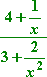This fraction is formed of two fractional expressions, one on top of the other. There are two methods for simplifying complex fractions. The first method is fairly obvious: find common denominators for the complex numerator and complex denominator, convert the complex numerator and complex denominator to their respective common denominators, combine everything in the complex numerator and in the complex denominator into single fractions, and then, once you've got one fraction (in the complex numerator) divided by another fraction (in the complex denominator), you flip-n-multiply. (Remember that, when you are dividing by a fraction, you flip the fraction and turn the division into multiplication.)

 This method looks like this: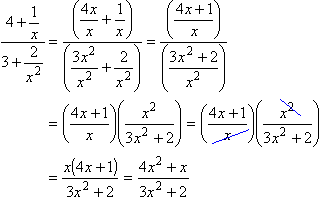Nothing cancels at this point, so this is the final answer.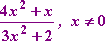(The "for x not equal to zero" part is because, in the original expression, "x = 0" would have caused division by zero in the complex fraction. Depending on your book and instructor, you may not need to account for this technicality . If you're not sure, ask now, before the test.)

The other method is to find one common denominator for all the fractions in the expression, and then multiply both the complex numerator and complex denominator by this expression. Then simplify.

 This method looks like this: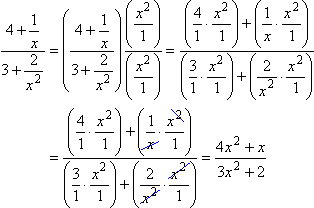By multiplying through, top and bottom, by the same thing, I was really just multiplying by 1. This is similar to multiplying the fraction 1/2 by 2/2 to convert it to 2/4. In my experience, books and teachers often use the first method, but students generally prefer the second method. When I was in school, I was taught the first method. As soon as I encountered the second method, I switched to it. In the remaining examples, I will demonstrate this second method, but you can use either method you prefer.

(If your text or instructor requires that you find the restrictions on the domains [the "x not equal to zero" part in the above example], you might find it helpful to use the "flip-n-multiply" method covered first, since this will give you the full fraction form of the denominator at some point in the computations.)

• Simplify the following expression:
•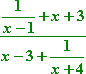Copyright © Elizabeth Stapel 2003-2011 All Rights Reserved

Can I start by hacking off the x's? Or lopping off the 3's? (Hint: No!) I can only cancel off factors, not terms, so I can't do any canceling yet. The first thing I'll do is find the LCM for this expression.

 The LCM (Least Common Multiple, or, for us older types, the LCD, Lowest Common Denominator) of the given denominators within this complex fraction is (x – 1)(x + 4), so I'll multiply through, top and bottom, by this expression:          (If you're not sure how I multiplied those factors to get the cubic results, review this lesson on multiplying polynomials.)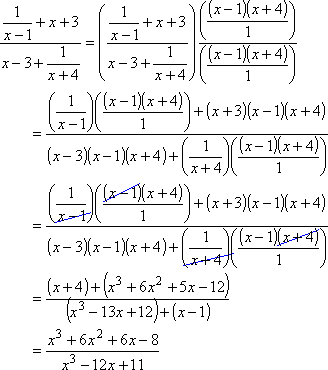Can I now cancel off the x3's? Or cancel the 6's into the 12? Can I go inside the adding and rip out parts of some of the terms? (Hint: No!) Nothing cancels, so this is the final answer: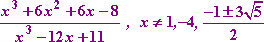(Why the restrictions? )